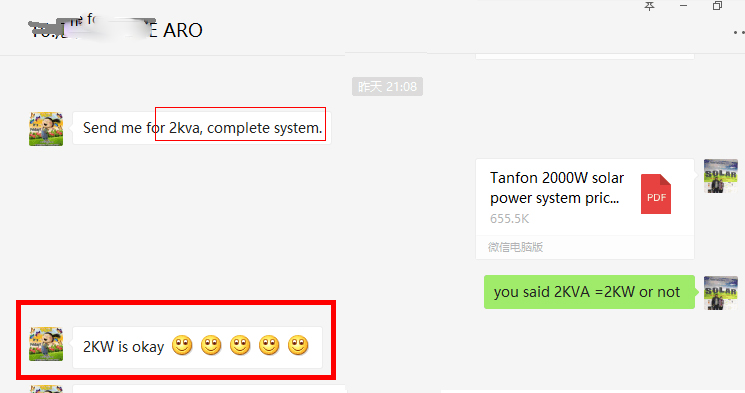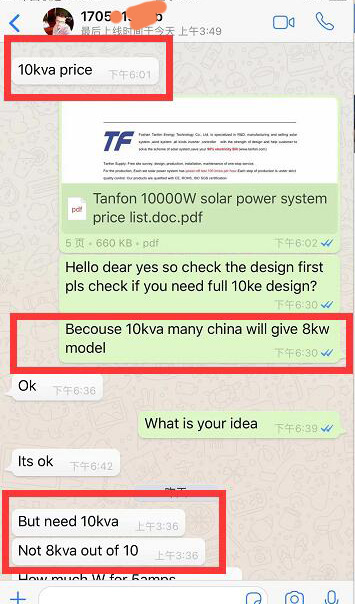Home > FAQ >

# What is the different between KVA and KW in solar power system?

0 Foshan Tanfon 2018-12-21 16:29:20

Have you ever noticed that with every appliance or piece of electrical machinery that you avail, they will always indicate their respective power ratings.

You will notice that some electrical equipment express their power ratings in kilowatts.

And some are expressed in KVA, Kilo Volt Amperes. Both values express power, but they are actually different. If you want to buy solar power system for home use, how to choose the right supplier?Many countries use KVA for power, such as Nigeria, the electrical equipment express the power ratings in KVA. But in China, we use KW, and actually 1000VA=700W (1KVA=0.7KW). All the quotes of TANFON are quoted in units of KW. For example, if you say 1KVA, the information we give you is 1KW.So if you buy our solar power system 10kw, it is equal to 14kva solar power system you buy from other supplier.

kW is the unit of active power. kVA is the unit of apparent power. Apparent power includes active power and reactive power. The relationship is:

apparent power ^ 2 = active power ^ 2 + reactive power ^ 2.

In order to simplify understanding of this formula, the concept of power factor ([email protected]) is now introduced. :

Active Power / Apparent Power = Power Factor.

The conversion between KW and KVA depends on the size of the power factor [email protected] -1<[email protected]<=1. (Normally, [email protected]=0.7)

KVA is known as the apparent power, while KW refers to the actual, or real power. KW is the amount of power capable of doing work, while only a portion of KVA is available to do work. KW is kilowatt, while KVA is kilo Volts Amperes.

In direct current circuits, KVA is equal to Kilowatt, because voltage and current do not get out of phase.

However, in AC circuits, voltage and current may get out of phase. Therefore, KW and KVA will differ depending on the Power Factor, or how much leading or lagging occurs.

Apparent power(KVA) and real power (KW) may differ in alternating current circuits. KW is simply the amount of actual power that does valid work.

Do you get that the different about KW and KVA now?

## TANFON 20KW Solar Power System 3 Phase for Factory Use

### latest products